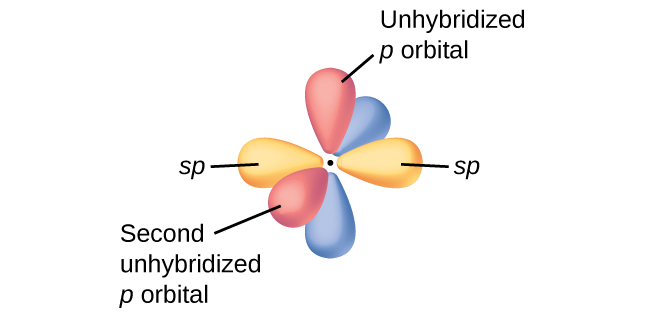# VSEPR theory tells that the valence electron pairs stay as far from each other as possible. For example, in BeCl_2 the two electron pairs of the two bonds are far apart so why we don't do this in the case of a double bond and assume it as a single bond ??

May 14, 2017

It's all a matter of orbitals.

#### Explanation:

VSEPR assumes that all the orbitals are equivalent hybridized atomic orbitals.

For example, the $\text{Be}$ atom in ${\text{BeCl}}_{2}$ uses equivalent atomic $\text{sp}$ orbitals to bond to the $\text{Cl}$ atoms.In ${\text{CO}}_{2}$, the $\text{C}$ atom uses two $\text{sp}$ hybrid orbitals for bonding.These $\text{sp}$ orbitals are on opposite sides of the carbon atom.

They are the orbitals that determine the shape of the molecule.

They are as far from each other as they can get, as predicted by VSEPR theory.

The unused "p" orbitals stay in place.When the $\text{O}$ atoms come in for bonding, they form σ bonds to the $\text{C}$ atom.

These bonds are at 180 ° to each other, as predicted by VSEPR theory.

The $\text{p}$ orbitals overlap to form π bonds between the $\text{C}$ and $\text{O}$ atoms, and this creates the $\text{C=O}$ double bonds.

Even though the electrons in the double bond repel each other, they are held in place by the attraction of the two nuclei.

The two electron pairs on each side of the carbon atom are at 180° to each other — as far apart as they can get.

For VSEPR purposes, the double bond is treated as if it were a single bond.